Learning library

For all the self-taught geeks out there, here is our content library with most of the learning materials we have produced throughout the years.

It makes sense to start learning by reading and watching videos about fundamentals and how things work.

Data Science and Machine Learning - 16 wks

Full-Stack Software Developer - 16w

Search from all Lessons

Social & live learning

The most efficient way to learn: Join a cohort with classmates just like you, live streams, impromptu coding sessions, live tutorials with real experts, and stay motivated.

← Back to Lessons
Edit on Github
Open in Collab

# Exploring Time Series

## Time series prediction in Python¶

To explain how we can do time series forecasting, we will use a famous data set on the evolution of the number of passengers on a famous American airline from 1949 to 1960.

### Step 1. Reading the data set¶

In :
import seaborn as sns


Out:
yearmonthpassengers
01949Jan112
11949Feb118
21949Mar132
31949Apr129
41949May121

The raw data set would be used to perform a usual Machine Learning process as we have seen in past modules. This time, we need to apply a transformation of it to generate a time series with two dimensions: the time dimension and the dimension of the data we want to analyze and predict. In this case the time dimension will be composed by the month (month) and the year (year) and the data we will observe over time will be the number of passengers (passengers).

In :
import pandas as pd

total_data["month"] = pd.to_datetime(total_data.month, format = "%b").dt.month
total_data["date"] = pd.to_datetime(total_data[["year", "month"]].assign(day = 1))
total_data = total_data.set_index("date")
ts = total_data["passengers"]

Out:
date
1949-01-01    112
1949-02-01    118
1949-03-01    132
1949-04-01    129
1949-05-01    121
Name: passengers, dtype: int64

Next, we will visualize the time series to perform a visual analysis of it:

In :
import matplotlib.pyplot as plt

fig, axis = plt.subplots(figsize = (10, 5))

sns.lineplot(data = ts)

plt.tight_layout()

plt.show()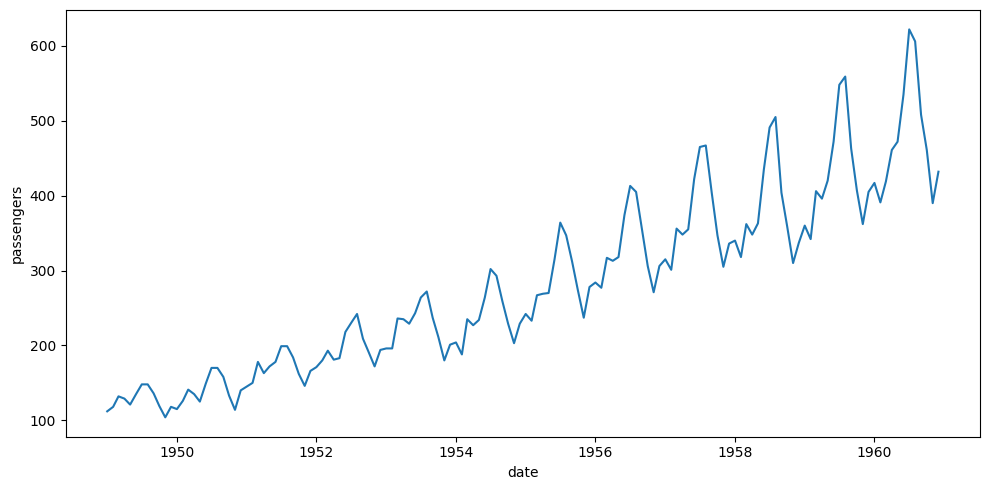### Step 2. Analysis of a time series¶

To analyze a time series, as we saw in the theory, we must study several parameters:

• Trend: An upward trend is apparent, indicating that the number of passengers has increased over time. This may be due to several factors: growth of the airline industry and provision of more resources to move passengers, price reduction, increased interest in air travel, and so on.
• Seasonality: There is some seasonality in the data, with certain months consistently having more flights than others. This could be due to seasonal demand (more people flying during the vacations, for example).
• Variability: There are some points of variability in the time series, especially between periods of increasing and decreasing demand.
• Outliers: Studying the trend and seasonality of the time series, no outliers are observed in the time series.
• Inflection points: Depending on the year, the increase in the number of passengers is not regular and sometimes there are variations in the slope; these are inflection points.

Through visual analysis we might be able to estimate these metrics by eye, but it is always better to orient the analysis to mathematical data. For the task of making predictions on time series and analyzing them, we will rely on the statsmodels library.

#### Decomposition of the series¶

The decomposition of a time series is a statistical process that separates a time series into several distinct elements. Each of these components represents a part of the underlying structure of the time series. Decomposing a time series can be very useful to better understand the data and make informed decisions when building forecasting models.

We use the seasonal_decompose function of the statsmodels library to decompose the time series into its trend, seasonality and residuals components.

In :
from statsmodels.tsa.seasonal import seasonal_decompose

decomposition = seasonal_decompose(ts, period = 12)
decomposition

Out:
<statsmodels.tsa.seasonal.DecomposeResult at 0x7f17b3115f10>

#### Trend analysis¶

Trend refers to the general direction in which the data is moving. To access its information we resort to the trend component of the decomposition.

In :
trend = decomposition.trend

fig, axis = plt.subplots(figsize = (10, 5))

sns.lineplot(data = ts)
sns.lineplot(data = trend)

plt.tight_layout()

plt.show()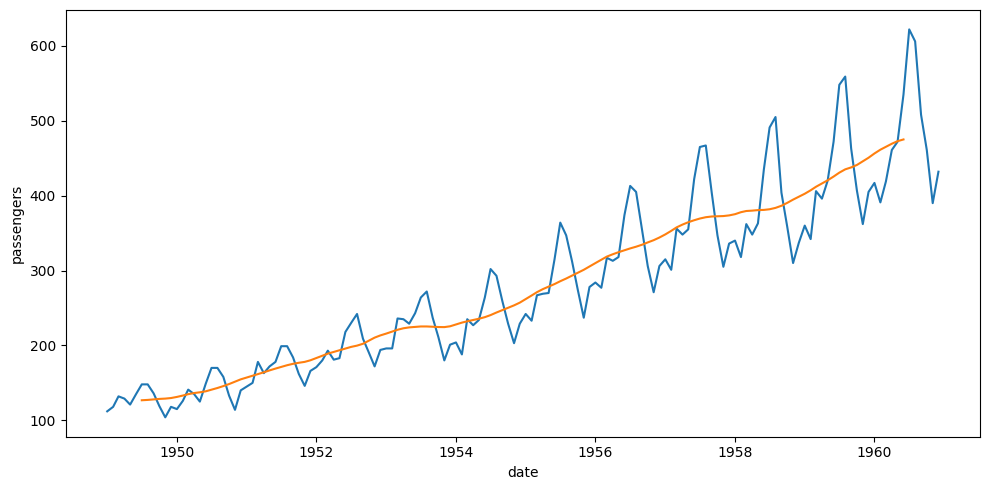This confirms what has been observed: a clear positive trend over the years.

#### Seasonality analysis¶

Trend refers to repetitive patterns in the data. To access its information we resort to the seasonal component of the decomposition.

In :
seasonal = decomposition.seasonal

fig, axis = plt.subplots(figsize = (10, 5))

sns.lineplot(data = ts)
sns.lineplot(data = seasonal)

plt.tight_layout()

plt.show()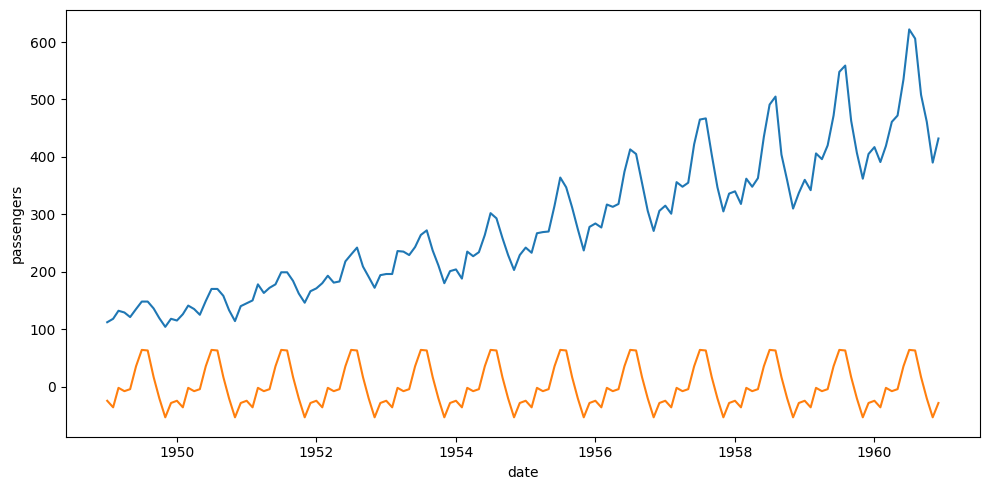To evaluate the stationarity of the time series we can apply the so-called Dickey-Fuller test, which is a hypothesis test in which the null hypothesis is that the series is stationary, and the alternative is that it is non-stationary:

In :
from statsmodels.tsa.stattools import adfuller

def test_stationarity(timeseries):
print("Dickey-Fuller test results:")
dftest = adfuller(timeseries, autolag = "AIC")
dfoutput = pd.Series(dftest[0:4], index = ["Test Statistic", "p-value", "#Lags Used", "Number of Observations Used"])
for key,value in dftest.items():
dfoutput["Critical Value (%s)"%key] = value
return dfoutput

test_stationarity(ts)

Dickey-Fuller test results:

Out:
Test Statistic                   0.815369
p-value                          0.991880
#Lags Used                      13.000000
Number of Observations Used    130.000000
Critical Value (1%)             -3.481682
Critical Value (5%)             -2.884042
Critical Value (10%)            -2.578770
dtype: float64

Here we can see that the p-value is greater than 0.05, this means that our null hypothesis will be rejected and we will take this series as non-stationary.

#### Analysis of variability¶

Variability involves the study of residuals: that is how the data fluctuate once trend and seasonality have been studied. To access their information we resort to the resid component of the decomposition.

In :
residual = decomposition.resid

fig, axis = plt.subplots(figsize = (10, 5))

sns.lineplot(data = ts)
sns.lineplot(data = residual)

plt.tight_layout()

plt.show()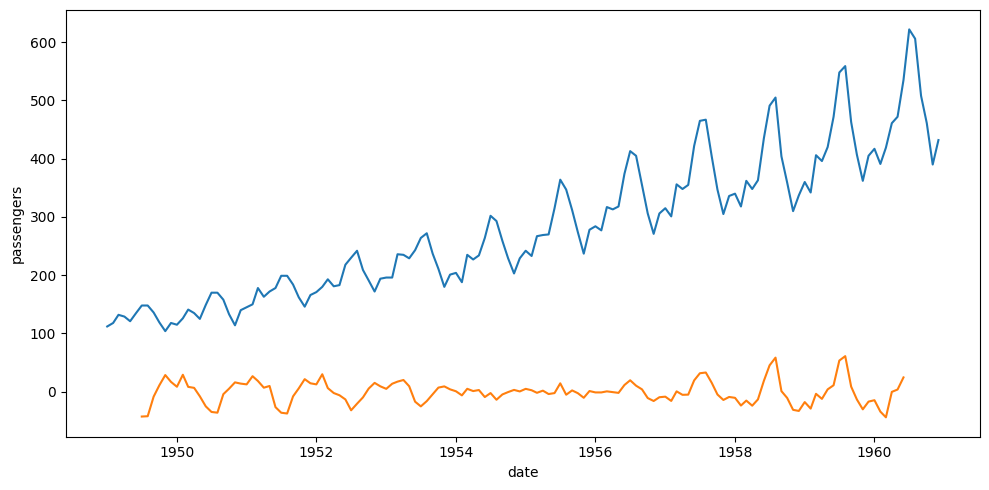This partly confirms what was observed, since the waste load is more noticeable at the beginning and end of the period studied.

#### Autocorrelation analysis¶

Autocorrelation is the correlation of a time series with a lagged copy of itself. This chart helps us to see if values in the time series are correlated with previous values.

In :
from statsmodels.graphics.tsaplots import plot_acf

plot_acf(ts)

plt.tight_layout()

plt.show()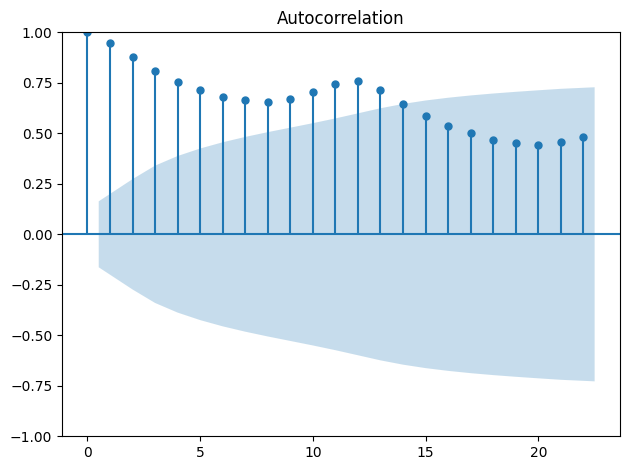There is a high correlation between the points and their delayed copies, which decreases over time.

### Step 3: Model training¶

An $ARIMA(p, d, q)$ model consists of three hyperparameters:

• p: The order of the autoregressive (AR) component.
• d: The degree of differencing needed to make the time series stationary.
• q: The order of the moving average (MA) component.

The study of these hyperparameters escapes our function since it is a purely mathematical-statistical analysis. Nowadays there are tools that make our life easier by estimating internally the most appropriate hyperparameters and generating the best possible model such as the pmdarima package and its auto_arima function. The only thing we have to consider is that in order to optimize its results to the maximum, we must transform the series into stationary, and as in the case of this series it is not, we must transform it:

In :
ts_stationary = ts.diff().dropna()

test_stationarity(ts_stationary)

Dickey-Fuller test results:

Out:
Test Statistic                  -2.829267
p-value                          0.054213
#Lags Used                      12.000000
Number of Observations Used    130.000000
Critical Value (1%)             -3.481682
Critical Value (5%)             -2.884042
Critical Value (10%)            -2.578770
dtype: float64

Now the series is, and we can apply the automatic ARIMA method:

In :
from pmdarima import auto_arima

model = auto_arima(ts_stationary, seasonal = True, trace = True, m = 12)

Performing stepwise search to minimize aic
ARIMA(2,0,2)(1,1,1) intercept   : AIC=inf, Time=1.57 sec
ARIMA(0,0,0)(0,1,0) intercept   : AIC=1033.479, Time=0.03 sec
ARIMA(1,0,0)(1,1,0) intercept   : AIC=1022.316, Time=0.23 sec
ARIMA(0,0,1)(0,1,1) intercept   : AIC=1022.905, Time=0.24 sec
ARIMA(0,0,0)(0,1,0)             : AIC=1031.508, Time=0.05 sec
ARIMA(1,0,0)(0,1,0) intercept   : AIC=1022.343, Time=0.07 sec
ARIMA(1,0,0)(2,1,0) intercept   : AIC=1021.137, Time=0.54 sec
ARIMA(1,0,0)(2,1,1) intercept   : AIC=inf, Time=2.57 sec
ARIMA(1,0,0)(1,1,1) intercept   : AIC=1022.411, Time=0.42 sec
ARIMA(0,0,0)(2,1,0) intercept   : AIC=1034.068, Time=0.39 sec
ARIMA(2,0,0)(2,1,0) intercept   : AIC=1023.008, Time=0.57 sec
ARIMA(1,0,1)(2,1,0) intercept   : AIC=1022.906, Time=0.63 sec
ARIMA(0,0,1)(2,1,0) intercept   : AIC=1021.017, Time=0.50 sec
ARIMA(0,0,1)(1,1,0) intercept   : AIC=1022.315, Time=0.15 sec
ARIMA(0,0,1)(2,1,1) intercept   : AIC=inf, Time=1.65 sec
ARIMA(0,0,1)(1,1,1) intercept   : AIC=1022.208, Time=0.49 sec
ARIMA(0,0,2)(2,1,0) intercept   : AIC=1022.997, Time=0.61 sec
ARIMA(1,0,2)(2,1,0) intercept   : AIC=1024.669, Time=1.21 sec
ARIMA(0,0,1)(2,1,0)             : AIC=1019.179, Time=0.27 sec
ARIMA(0,0,1)(1,1,0)             : AIC=1020.426, Time=0.12 sec
ARIMA(0,0,1)(2,1,1)             : AIC=inf, Time=1.31 sec
ARIMA(0,0,1)(1,1,1)             : AIC=1020.328, Time=0.51 sec
ARIMA(0,0,0)(2,1,0)             : AIC=1032.121, Time=0.17 sec
ARIMA(1,0,1)(2,1,0)             : AIC=1021.033, Time=0.45 sec
ARIMA(0,0,2)(2,1,0)             : AIC=1021.148, Time=0.27 sec
ARIMA(1,0,0)(2,1,0)             : AIC=1019.240, Time=0.24 sec
ARIMA(1,0,2)(2,1,0)             : AIC=1022.806, Time=0.63 sec

Best model:  ARIMA(0,0,1)(2,1,0)
Total fit time: 15.917 seconds


As we can see, the function searches the possible solution space to estimate the best parameters. In this case we would have an $ARIMA(0, 0, 1)$. The model returned by this function is fully usable, like any other we have seen, and its summary() function returns statistical and performance information that is of great value:

In :
model.summary()

Out:
Dep. Variable: No. Observations: y 143 SARIMAX(0, 0, 1)x(2, 1, [], 12) -505.589 Thu, 03 Aug 2023 1019.179 16:30:12 1030.680 02-01-1949 1023.852 - 12-01-1960 opg
coef std err z P>|z| [0.025 0.975] -0.3634 0.074 -4.945 0.000 -0.508 -0.219 -0.1239 0.090 -1.371 0.170 -0.301 0.053 0.1911 0.107 1.783 0.075 -0.019 0.401 130.4480 15.527 8.402 0.000 100.016 160.880
 Ljung-Box (L1) (Q): Jarque-Bera (JB): 0.01 4.59 0.92 0.1 2.7 0.15 0 3.87

Warnings:
 Covariance matrix calculated using the outer product of gradients (complex-step).

#### Step 3: Model prediction¶

Once the model has been trained, it can be used to predict into the future (we will predict the next 10 months)

In :
forecast = model.predict(10)
forecast

Out:
1961-01-01    19.346932
1961-02-01   -24.244912
1961-03-01    36.280007
1961-04-01    36.323602
1961-05-01    14.329657
1961-06-01    57.816446
1961-07-01    89.458676
1961-08-01   -13.228998
1961-09-01   -96.797005
1961-10-01   -50.216336
Freq: MS, dtype: float64
In :
import matplotlib.pyplot as plt

fig, axis = plt.subplots(figsize = (10, 5))

sns.lineplot(data = ts_stationary)
sns.lineplot(data = forecast, c = "green")

plt.tight_layout()

plt.show()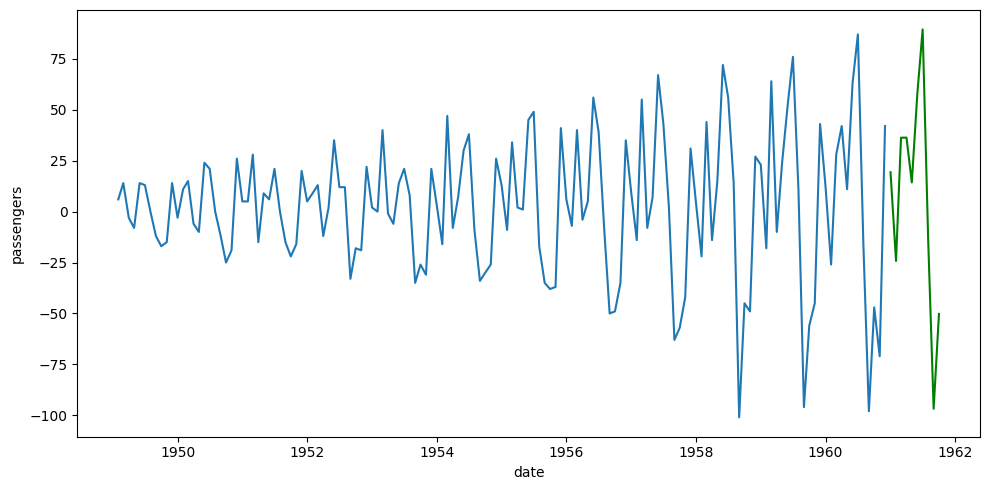Our model is now able to make forward predictions on our stationary series.